• 从3d max导出的fpx模型文件导入UE4时要注意很多，不然当模型导入UE4后就会出现很多问题，如坐标轴不理想，坐标原点偏离物体不在物体本身，这样就会导致在UE4 中移动物体的麻烦，对物体进行缩放，旋转也会很麻烦。...
从3d max导出的fpx模型文件导入UE4时要注意很多，不然当模型导入UE4后就会出现很多问题，如坐标轴不理想，坐标原点偏离物体不在物体本身，这样就会导致在UE4 中移动物体的麻烦，对物体进行缩放，旋转也会很麻烦。本文介绍一下解决这种问题的一种办法。 第一步：把出现问题的模型从新用3d max打开或者3d max直接对原有的模型进行编辑，如果是在一个场景中选择一个模型的话，选中这个模型之后先孤立这个模型然后会这个模型进行编辑。 第二步：如果是修正坐标轴的话，选中目标后点击编辑框里的层次选项，点击调整轴立面的仅影响轴。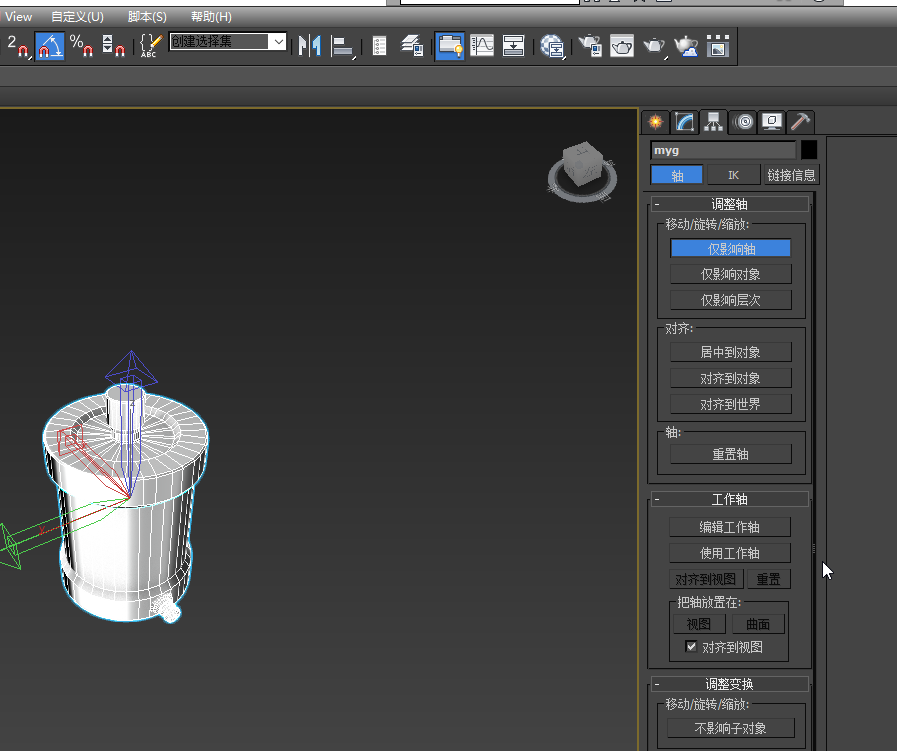然后鼠标点击右键，选择移动，就可以修改坐标轴了，可以吧坐标轴修改到理想的位置。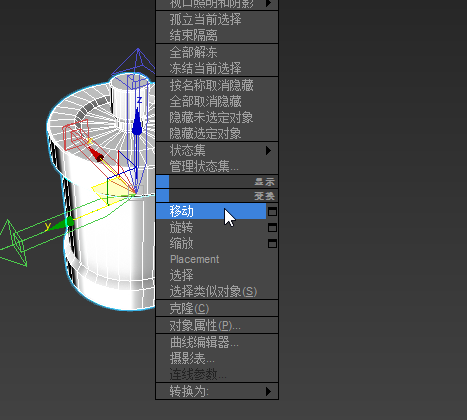当把坐标轴调整到理想位置后，把仅影响轴这个选项取消后就可以调整原点坐标，把 下面的x,y,z坐标置零就可以了。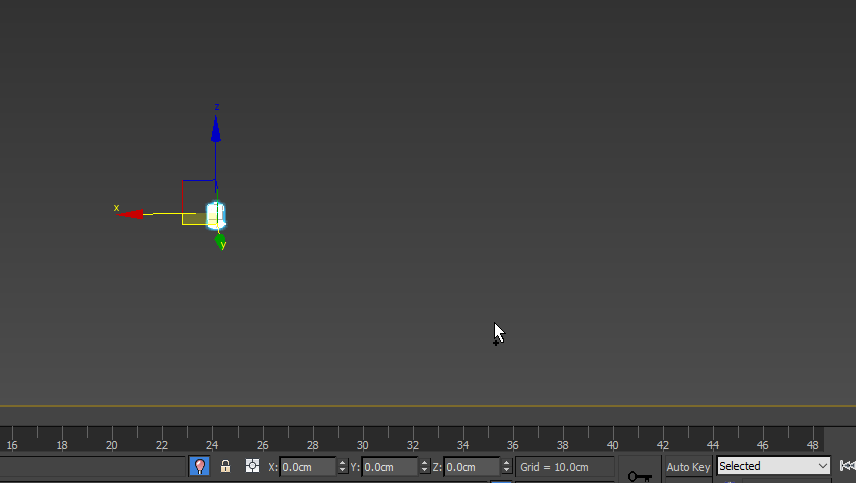然后把调整好的模型直接导出fpx文件就可以了，导出的时候命名文件不要用中文，不然很有可能出现未知错误这样调整好的模型导入UE4就不会出现坐标原点和坐标轴等问题了。
展开全文• 解决gltf模型位置偏离原点导致加载找不到的问题 cesium加载gltf模型，由于给我的gltf模型的位置偏离原点太多，导致加载进去后找不到模型在哪个角落。 var position = Cesium.Cartesian3.fromDegrees(113.2, 23.18, 0...
解决gltf模型位置偏离原点导致加载找不到的问题
cesium加载gltf模型，由于给我的gltf模型的位置偏离原点太多，导致加载进去后找不到模型在哪个角落。
var position = Cesium.Cartesian3.fromDegrees(113.2, 23.18, 0);
var pitch = 0;
var roll = 0;
position,
hpr
);
name: url,
position: position,
orientation: orientation,
model: {
uri: url,
minimumPixelSize: 128,
maximumScale: 20000,
},
});

又是用的nginx，将gltf及其bin文件放进nginx，作为资源服务器。 查看entity的position，这个就是创建时的选项position。 更改gltf文件，文件末尾指定其bin文件。translation属性是每个部件的位置，修改translation的值，这是个数组，修改之后，使其接近原点，就可以在cesium设置的位置看到模型了。 坑人，给个偏离原点四万多的gltf模型，害我找半天问题。
展开全文javascript
• ggplot2绘图结果往往X轴和Y轴的截距交点往往不是原点：譬如下图y轴就不是起始于0 调整坐标起始位点可以利用scale_y_continuous(expand = c(0, 0))或者scale_x_continuous(expand = c(0, 0)) expand的解释如下 ...

ggplot2绘图结果往往X轴和Y轴的截距交点往往不是原点：譬如下图y轴就不是起始于0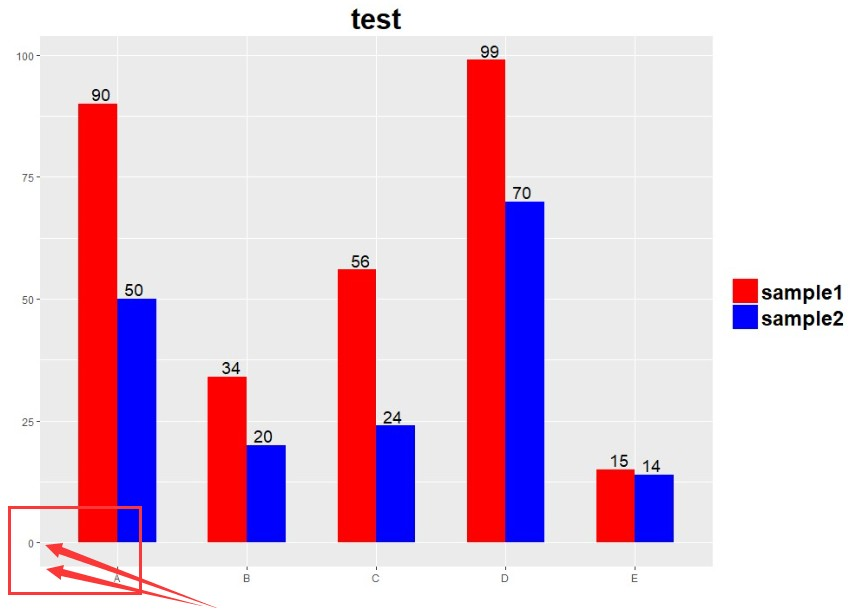调整坐标起始位点可以利用scale_y_continuous(expand = c(0, 0))或者scale_x_continuous(expand = c(0, 0))
expand的解释如下
expand A numeric vector of length two giving multiplicative and additive expansion constants. These constants ensure that the data is placed some distance away from the axes. The defaults are c(0.05, 0) for continuous variables, and c(0, 0.6)for discrete variables.   连续性和离散型都涉及，默认值不同，离散型scale_y_discrete 或者scale_x_discrete，这里针对连续性进行介绍：
参考画图数据和代码   http://www.omicsclass.com/article/92
修改y轴连续性坐标起点：y = p + scale_y_continuous(expand=c(0,0))

如果针对x离散型的调整呢?尝试调整数，使柱子紧贴Y轴：x = p + scale_x_discrete(expand=c(0,0))

转载于:https://www.cnblogs.com/xiaofeiIDO/p/10858410.html
展开全文• ## 支持向量机

千次阅读 多人点赞 2016-07-18 23:04:15
是将平面平移到坐标原点所需距离（所以 b = 0 b=0 时，平面过原点） 向量的模 给定一个向量 w = ( w 1 , w 2 , w 3 ) w = (w_1,w_2,w_3) , 则 | w | = s q r t ( w 2 1 + w 2 2 + w 2 3 ) |w| = sqrt...
支持向量机

支持向量机
点到平面的距离
平面的一般式方程向量的模向量的内积点到平面的距离 最优间隔分类器与支持向量
函数间隔和几何间隔如何确定这个超平面最大间隔划分超平面 对偶问题
对偶问题转化对偶问题求解 拉格朗日乘子法和KKT条件
拉格朗日乘子法等式约束问题等式约束问题的合理性拉格朗日乘子法不等式约束问题KKT条件不等式约束问题的合理性 核函数
特征空间的隐式映射核函数有效性判定
半正定矩阵问题描述与分析有效地核函数定义  软间隔与正则化SMO算法详解
两个Lagrange乘子的优化问题 支持向量回归核方法

点到平面的距离

平面的一般式方程

w1x1+w2x2+w3x3+b=wTx+b=0

w_1x_1+w_2x_2+w_3x_3+b=w^Tx+b=0
其中

w=(w1,w2,w3)
$w = (w_1,w_2,w_3)$ 是平面的法向量，

b
$b$ 是将平面平移到坐标原点所需距离（所以 b=0$b=0$ 时，平面过原点）

向量的模
给定一个向量

w=(w1,w2,w3)
$w = (w_1,w_2,w_3)$, 则

|w|=sqrt(w21+w22+w23)
$|w| = sqrt(w_1^2+ w_2^2+ w_3 ^2)$
向量的内积
给定两个向量

w=(w1,w2,w3)
$w = (w_1,w_2,w_3)$ 和

x=(x1,x2,x3)
$x = (x_1,x_2,x_3)$ 则他们的内积是

wx=w1x1+w2x2+w3x3

wx = w_1x_1+w_2x_2+w_3x_3

点到平面的距离
!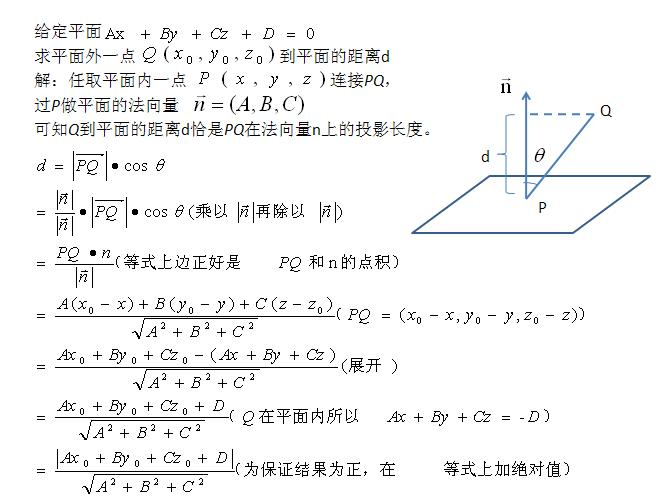最优间隔分类器与支持向量
函数间隔和几何间隔

令划分超平面的线性方程为

wTx+b=0

w^Tx+b=0
假设超平面能正确分类，当

wTx+b>0
$w^Tx+b>0$ 的点对应

y=1
$y=1$ 的数据点，

wTx+b<0
$w^Tx+b<0$ 的点对应

y=−1
$y=-1$ 的点。
给定一个训练样本

(x(i),y(i))
$(x^{(i)},y^{(i)})$。我们定义函数间隔如下：

γ(i)=y(i)(wTx(i)+b)

{\over{  \gamma}} ^{(i)}= {y^{\left( i \right)}}\left( {{w^T}{x^{\left( i \right)}} + b} \right)
实际上

γ(i)=∣∣wTx(i)+b∣∣

{\over{  \gamma}} ^{(i)}= {{\left| {{w^T}x^{(i)}  + b} \right|}}

为了使函数间隔最大（更大的信心确定该例是正例还是反例），当

y(i)=1
$y^{(i)}=1$ 时，

wTx(i)+b
${{w^T}x^{(i)} + b}$ 应该是个大正数，反之是个大负数。因此函数间隔代表了我们认为特征是正例还是反例的确信度。
继续考虑

w
$w$ 和 b$b$，如果同时加大

w
$w$ 和 b$b$，比如在

wTx(i)+b
${{w^T}x^{(i)} + b}$ 前面乘个系数比如2，那么所有点的函数间隔都会增大二倍，这个对求解问题来说不应该有影响，因为我们要求解的是

wTx(i)+b=0
${{w^T}x^{(i)} + b}=0$ ，同时扩大

w
$w$ 和 b$b$对结果是无影响的。这样，我们为了限制

w
$w$ 和 b$b$，可能需要加入归一化条件
现在我们定义全局样本上的函数间隔

γ=mini=1,...,mγ(i)

{\over{  \gamma}} = \mathop {\min }\limits_{i=1,...,m}{\over{  \gamma}} ^{(i)}

说白了就是在训练样本上分类正例和负例确信度最小那个函数间隔。
样本空间任意点

x(i)
$x^{(i)}$ 到超平面的几何间隔为

r(i)=∣∣wTx(i)+b∣∣∥w∥=γ(i)∥w∥

r^{(i)} = {{\left| {{w^T}x^{(i)}  + b} \right|} \over {\left\| w \right\|}}= {{\over{  \gamma}} ^{(i)} \over {\left\| w \right\|}}
函数间隔归一化结果就是几何间隔。同时扩大

w
$w$ 和 b$b$，

w
$w$ 扩大几倍，∥w∥${\left\| w \right\|}$　就扩大几倍，结果无影响。
定义全局的几何间隔

r=mini=1,...,mr(i)

r= \mathop {\min }\limits_{i=1,...,m}r^{(i)}

分类学习最基本的想法就是基于给定训练集在样本空间中找到一个划分超平面，将不同的类别样本分开。但满足这种要求的划分超平面有很多。  !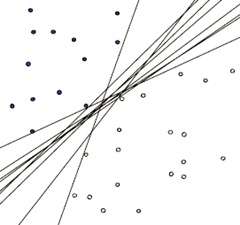如何确定这个超平面
直观上讲，这个超平面应该是最适合分开两类数据的直线。而判定“最适合”的标准就是寻找一个超平面，使得离超平面比较近的点能有更大的间距。也就是我们不考虑所有的点都必须远离超平面，我们关心求得的超平面能够让所有点中离它最近的点具有最大间距。
形式化表示为

maxr,w,bγ∥w∥s.t.y(i)(wTx(i)+b)≥γ,i=1,2,...,m

\mathop {\max }\limits_{r,w,b}{{\over{  \gamma}}\over {\left\| w \right\|}}\\
s.t.{\rm{  }}{y^{(i)}}\left( {{w^T}{x^{(i)}} + b} \right) \ge{\over{  \gamma}} {\rm{, }}i = 1,2,...,m

前面说到同时扩大

w
$w$ 和 b$b$ 对结果没有影响，但我们最后要求的仍然是

w
$w$ 和 b$b$ 的确定值。因此，我们需要对

γ
$\over \gamma$ 做一些限制，以保证我们解是唯一的。这里为了简便我们取

γ=1
${\over{ \gamma}} =1$ 。这样的意义是将全局的函数间隔定义为1。即将离超平面最近的点的距离定义为

1∥w∥
$1\over {\left\| w \right\|}$  即令

{wTxi+b≥+1,yi=+1wTxi+b≤−1,yi=−1

\left\{ \matrix{
{w^T}{x_i} + b \ge  + 1{\rm{,}}{{\rm{y}}_i} =  + 1\cr
{w^T}{x_i} + b \le  - 1{\rm{,}}{{\rm{y}}_i} =  - 1 \cr}  \right.
如下图所示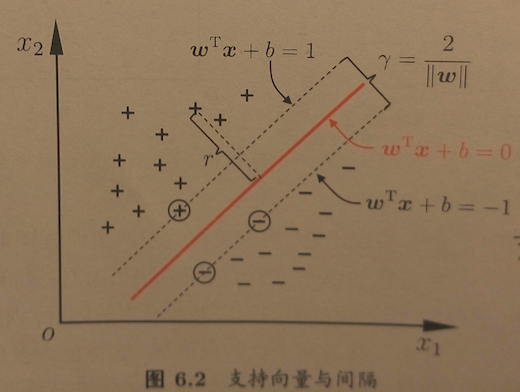上式使得等号成立的几个样本点被称为支持向量
两个异类支持向量到超平面距离之和为：

γ=2∥w∥

\gamma  = {2 \over {\left\| w \right\|}}
被称为
间隔

最大间隔划分超平面
欲找到最大间隔的划分超平面，我们得到改写后的目标函数为

maxw,b2∥w∥s.t.yi(wTxi+b)≥1,i=1,2,...,m

\eqalign{
& \mathop {\max }\limits_{w,b} {2 \over {\left\| w \right\|}}  \cr
& s.t.{\rm{  }}{y_i}\left( {{w^T}{x_i} + b} \right) \ge 1{\rm{, }}i = 1,2,...,m \cr}

我们在优化时喜欢求最小值，将上式转化正等价的求最小值如下

minw,b12∥w∥2s.t.yi(wTxi+b)≥1,i=1,2,...,m

\eqalign{
& \mathop {\min }\limits_{w,b} {1 \over 2}{\left\| w \right\|^2}  \cr
& s.t.{\rm{  }}{y_i}\left( {{w^T}{x_i} + b} \right) \ge 1{\rm{, }}i = 1,2,...,m \cr}
这就是支持向量机的
基本型

对偶问题

对偶问题转化
上式是一个凸二次规划问题，我们可以使用拉格朗日乘数法进行优化，对每个约束添加拉格朗日乘子

αi≥0
$\alpha_i \ge 0$。则问题的拉格朗日函数可写为

L(w,b,α)=12∥w∥2+∑i=1mαi(1−yi(wTxi+b))

L(w,b,\alpha ) = {1 \over 2}{\left\| w \right\|^2} + \sum\limits_{i = 1}^m {{\alpha _i}\left( {1 - {y_i}\left( {{w^T}{x_i} + b} \right)} \right)}
式中

αi
$\alpha_i$是拉格朗日乘子.
我们令

θ(w)=maxαi≥0L(w,b,α)

\theta (w) = \mathop {\max }\limits_{{\alpha _i} \ge 0} L(w,b,\alpha )
如果约束条件都满足，

θ(w)
$\theta (w)$ 的最优值就是

12∥w∥2
${1 \over 2}{\left\| w \right\|^2}$，和目标函数一样。

因此我们可以直接求

θ(w)
$\theta (w)$ 的最小值，等价于求原目标函数。因此目标函数变成如下

minw,bθ(w)=minw,bmaxαi≥0L(w,b,α)=p∗

\mathop {\min }\limits_{w,b} \theta (w) = \mathop {\min }\limits_{w,b} \mathop {\max }\limits_{{\alpha _i} \ge 0} L(w,b,\alpha )=p^*

将求最大值和最小值交换位置，得到原始问题的对偶问题

maxαi≥0minw,bL(w,b,α)=d∗

\mathop {\max }\limits_{{\alpha _i} \ge 0} \mathop {\min }\limits_{w,b} L(w,b,\alpha ) = {d^*}

满足

d∗≤p∗

d^* \le p^*
在满足某些条件的情况下(满足KKT条件)，这两者相等，这个时候就可以通过求解对偶问题来间接地求解原始问题。

对偶问题求解
分别求

L
$L$ 对 w,b$w,b$ 的导数，并令其为0，得如下结果

∂L∂w=0⇒w=∑i=1mαiyixi∂L∂b=0⇒∑i=1mαiyi=0

\eqalign{
& {{\partial L} \over {\partial w}} = 0 \Rightarrow w = \sum\limits_{i = 1}^m {{\alpha _i}{y_i}{x_i}}   \cr
& {{\partial L} \over {\partial b}} = 0 \Rightarrow \sum\limits_{i = 1}^m {{\alpha _i}{y_i}}  = 0 \cr}

带入

L(w,b,α)
$L(w,b,\alpha )$ 得到

minw,bL(w,b,α)
$\mathop {\min }\limits_{w,b} L(w,b,\alpha )$

minw,bL(w,b,α)=12∑i,j=1mαiαjyiyjxTixj−∑i,j=1mαiαjyiyjxTixj−b∑i=1mαiyi+∑i=1mαi=∑i=1mαi−12∑i,j=1mαiαjyiyjxTixj

\eqalign{
& \mathop {\min }\limits_{w,b} L(w,b,\alpha ) = {1 \over 2}\sum\limits_{i,j = 1}^m {{\alpha _i}{\alpha _j}{y_i}{y_j}x_i^T{x_j} - } \sum\limits_{i,j = 1}^m {{\alpha _i}{\alpha _j}{y_i}{y_j}x_i^T{x_j}}   \cr
&  - b\sum\limits_{i = 1}^m {{\alpha _i}{y_i}}  + \sum\limits_{i = 1}^m {{\alpha _i}}  = \sum\limits_{i = 1}^m {{\alpha _i}}  - {1 \over 2}\sum\limits_{i,j = 1}^m {{\alpha _i}{\alpha _j}{y_i}{y_j}x_i^T{x_j}}  \cr}

然后求最大值，从上面的式子得到对偶问题

maxαi≥0∑i=1mαi−12∑i,j=1mαiαjyiyjxTixjs.t.∑i=1mαiyi=0,αi≥0,i=1,2,...,m

\eqalign{
& \mathop {\max }\limits_{{\alpha _i} \ge 0} \sum\limits_{i = 1}^m {{\alpha _i}}  - {1 \over 2}\sum\limits_{i,j = 1}^m {{\alpha _i}{\alpha _j}{y_i}{y_j}x_i^T{x_j}}   \cr
& s.t.{\rm{  }}\sum\limits_{i = 1}^m {{\alpha _i}} {y_i} = 0{\rm{,  }}{\alpha _i} \ge 0{\rm{,   }}i = 1,2,...,m \cr}

上式是关于

α
$\alpha$ 的式子，如果能求出

α
$\alpha$ ，则通过

w=∑i=1mαiyixi
$w = \sum\limits_{i = 1}^m {{\alpha _i}{y_i}{x_i}}$ 可以求出

w
$w$，再根据前面函数距离等于1的假设求出 b$b$。
得到模型

f(x)=wTx+b=∑i=1mαiyixTix+b=∑i=1mαiyi⟨xi,x⟩+b

f\left( x \right) = {w^T}x + b = \sum\limits_{i = 1}^m {{\alpha _i}{y_i}x_i^Tx}  + b = \sum\limits_{i = 1}^m {{\alpha _i}{y_i}} \left\langle {{x_i},x} \right\rangle  + b
对于新点

x
$x$ 的预测，只需计算它与训练数据点的内积即可。且所有非支持向量所对应的系数 α$α$ 都等于零(这里为满足

maxαi≥0L(w,b,α)
$\mathop {\max }\limits_{{\alpha _i} \ge 0} L(w,b,\alpha )$ 最大化必须等于0)，因此对于内积计算只针对支持向量即可。

拉格朗日乘子法和KKT条件

拉格朗日乘子法和KKT(Karush-Kuhn-Tucker)条件是求解约束优化问题的重要方法，在有等式约束时使用拉格朗日乘子法，在有不等约束时使用KKT条件。
前提：只有当目标函数为凸函数时，使用这两种方法才保证求得的是最优解。
最优化问题分类:  1). 如果目标函数和约束条件都为变量的线性函数, 称该最优化问题为线性规划;  2). 如果目标函数为变量的二次函数, 约束条件为变量的线性函数, 称该最优化问题为二次规划;  3). 如果目标函数或者约束条件为变量的非线性函数, 称该最优化问题为非线性规划.
拉格朗日乘子法–等式约束问题
对于等式约束问题

x⋆=argminxf(x)subject to hi(x)=0,∀i=1,..,m

\mathbf{x^\star} = \underset{\mathbf{x}}{\textrm{argmin}} f(\mathbf{x})
\\\textrm{subject to } h_i(\mathbf{x}) = 0, \forall i=1,..,m
利用拉格朗日乘子法，原问题转换为

x⋆=argmin xL(x,λ)=argmin xf(x)+∑mi=1λihi(x),

\mathbf{x^\star} = \underset{\mathbf{x}}{\textrm{argmin }} L(\mathbf{x}, \mathbf{\lambda}) = \underset{\mathbf{x}}{\textrm{argmin }} f(\mathbf{x})  + \underset{i=1}{\overset{m}{\sum}} \lambda_i h_i(\mathbf{x}),
其中

λi≠0
$\lambda_{i}\ne{0}$，称为拉格朗日乘子，这样我们有了

k+m
$k+m$ 个变量。

通过对

x
$x$ 求偏导，找最优解，得到最优的条件
∇xL(x,λ)=∇xf(x)+∑iλi∇xhi(x)=0\nabla_{\mathbf{x}}L(\mathbf{x}, \lambda) = \nabla_{\mathbf{x}}f(\mathbf{x}) + \sum_i\lambda_i \nabla_{\mathbf{x}}h_i(\mathbf{x}) = 0
我们得到了

k
$k$ 个方程式。进一步对拉格朗日乘子求偏导，得到另外 m$m$ 个方程式

∂L(x,λ)∂λi=hi(x)=0,

\frac{\partial L(\mathbf{x}, \lambda)}{\partial \lambda_i} = h_i(\mathbf{x}) = 0,

这里通过强制偏导数为零，我们同样可以限制可能的解满足原始约束条件。现在变量数与方程数相同，使得方程解唯一。
等式约束问题的合理性
举个二维最优化的例子

minf(x,y)s.t.g(x,y)=c

\min\;f(x,y)\\s.t.\;g(x,y)=c

我们可以画图来辅助思考  !Alt text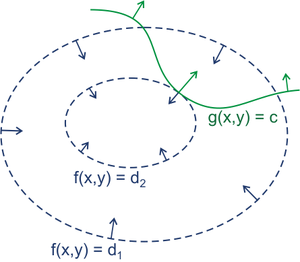蓝线是

f(x,y)
$f(x,y)$ 的等高线。箭头表示斜率，和等高线的法线平行。从梯度的方向上来看，显然有

d1>d2
$d_1>d_2$。  绿线标出的是约束

g(x,y)=c
$g(x,y)=c$ 的点的轨迹，也就是说，只要正好落在这条绿线上的点才可能是满足要求的点。
最小值点应该在哪里呢？显然应该是在

f(x,y)
$f(x,y)$ 的等高线正好和约束线相切的位置，因为如果只是相交意味着肯定还存在其它的等高线在该条等高线的内部或者外部，使得新的等高线与目标函数的交点的值更大或者更小，只有到等高线与目标函数的曲线相切的时候，可能取得最优值。
很容易看出来，要想让目标函数

f(x,y)
$f(x,y)$ 的等高线和约束相切，则他们切点的梯度一定在一条直线上。即在最优解处，

f
$f$ 和 g$g$ 的斜率平行。  因此，我们通过观察可以得到优化取到最小值时满足

▽[f(x,y)+λ(g(x,y)−c)]=0λ≠0

\bigtriangledown[f(x,y)+\lambda(g(x,y)-c)]=0\;\;\;\;\lambda\ne{0}

这个结果正好等价于方程

F(x,y)=f(x,y)+λ(g(x,y)−c)
$F(x,y)=f(x,y)+\lambda(g(x,y)-c)$ 对

x
$x$ 求偏导。方程 F(x,y)$F(x,y)$ 在达到极值时与

f(x,y)
$f(x,y)$ 相等，因为

F(x,y)
$F(x,y)$达到极值时

g(x,y)−c
$g(x,y)−c$总等于零。
拉格朗日乘子法–不等式约束问题
当同时存在不等式约束时，新的问题描述为

x∗=argminxf(x)

{x^*} = \underset{{x}}{\textrm{argmin}} f({x})

subject to hi(x)=0,∀i=1,..,msubject to gi(x)≤0,∀i=1,..,n

\textrm{subject to } h_i({x}) = 0, \forall i=1,..,m
\\\textrm{subject to } g_i({x}) \le 0, \forall i=1,..,n

此时不等式约束下的最优化问题描述为

x∗=argmin xL(x,λ,μ)=argmin xf(x)+∑mi=1λihi(x)+∑ni=1μigi(x)

{x^*} = \underset{{x}}{\textrm{argmin }} L({x}, {\lambda}, {\mu}) = \underset{{x}}{\textrm{argmin }} f({x})  + \underset{i=1}{\overset{m}{\sum}} \lambda_i h_i({x}) + \underset{i=1}{\overset{n}{\sum}} \mu_i g_i({x})

其中

L(x,λ,μ)
$L({x}, {\lambda}, {\mu})$ 为拉格朗日函数，

λi
$\lambda_i$ 对应等式约束系数，

μk
$\mu_{k}$ 对应不等式约束系数。现在总共有

k+m+n
$k+m+n$个变量。
跟等式约束一样，我们想通过对

x
$x$ 与 λ$\lambda$ 求偏导，得到

k+m
$k+m$ 个方程式。接下来的问题是怎么通过不等式约束得到更多的

n
$n$ 个方程式。

对于不等式约束，当极值点存在 gi(x∗)<0$g_i({x^*}) \lt 0$ 区域，那么这个约束不影响极值的取值，等同于没有约束。因此它的系数

μi
$\mu_i$ 可以设置为0。另一方面，如果极值在约束边界上，此时，

gi(x∗)=0
$g_i({x^*}) = 0$。如下图所示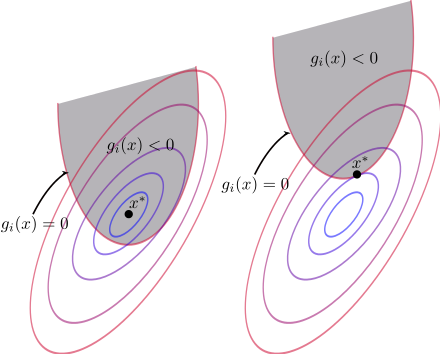综合可得到

μigi(x)=0

\mu_i g_i({x}) = 0
为问题的必要条件。至此，我们得到了

n
$n$ 个方程式通过不等式约束。系数 λi$\lambda_i$ 可以为任意值，没有符号的限制。然而

μk≥0
$\mu_{k} \ge 0$ ，下图解释说明。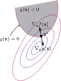假设

x∗
$x^*$ 在

gi(x)=0
$g_i({x})=0$ 区域内，那么

μi
$\mu_{i}$ 可以不为零。

{x^*} = \underset{{x}}{\textrm{argmin }} f({x})  + \mu_i g_i({x})\\0 = \nabla f({x})  + \mu_i \nabla g_i({x})\\\begin{equation}\mu_i = -\frac{\nabla f({x})}{\nabla g_i({x})}\label{eq:signofmu}\end{equation}
{x^*} = \underset{{x}}{\textrm{argmin }} f({x})  + \mu_i g_i({x})
\\0 = \nabla f({x})  + \mu_i \nabla g_i({x})
\\\begin{equation}\mu_i = -\frac{\nabla f({x})}{\nabla g_i({x})}\label{eq:signofmu}\end{equation}

在

x∗
$x^*$ 点处，

f(x)
$f(x)$ 与

gi(x)
$g_i(x)$ 的梯度方向像相反。所以

μi≥0
$\mu_{i} \ge 0$
KKT条件
对于一个标准形式的最优化数学模型

x∗=argminxf(x)

{x^*} = \underset{{x}}{\textrm{argmin}} f({x})

subject to hi(x)=0,∀i=1,..,msubject to gi(x)≤0,∀i=1,..,n

\textrm{subject to } h_i({x}) = 0, \forall i=1,..,m
\\\textrm{subject to } g_i({x}) \le 0, \forall i=1,..,n

KKT条件是一个非线性规划问题能有最优化解法的必要和充分条件。KKT条件就是指上面最优化问题中的最小点

x∗
$x^*$ 必须满足下面的条件：

⎧⎩⎨⎪⎪⎪⎪⎪⎪⎪⎪⎪⎪⎪⎪⎪⎪⎪⎪⎪⎪⎪⎪⎪⎪⎪⎪⎪⎪⎪⎪⎪⎪⎪⎪⎪⎪⎪⎪⎪⎪Stationarity:Primalfeasibility:Dualfeasibility:Complementaryslackness:0=∇f(x)+∑iλi∇hi(x)+∑iμi∇gi(x)hi(x)=0,∀i=1,..,mgi(x)≤0,∀i=1,..,nμi≥0,∀i=1,..,nμigi(x)=0,∀i=1,..,m

\left\{\matrix{
Stationarity: \\&
0 = \nabla f({x}) + \sum_i\lambda_i \nabla_{}h_i(\mathbf{x}) +  \sum_i{\mu_i \nabla g_i({x}) }\\
Primal feasibility:\\&
h_i({x}) = 0, \forall i=1,..,m\\&
g_i({x}) \le 0, \forall i=1,..,n\\
Dual feasibility:\\&
\mu_{i}\ge{0}, \forall i=1,..,n\\
Complementary slackness:\\&
\mu_i g_i({x}) = 0, \forall i=1,..,m
}  \right.

不等式约束问题的合理性
为什么可对偶问题求解

L(x,μ)=f(x)+∑k=1qμkgk(x)μk≥0gk(x)≤0⎫⎭⎬⎪⎪⎪⎪⎪⎪⎪⎪

\left.\begin{matrix}L(x,\mu)=f(x)+\sum_{k=1}^q\mu_{k}g_{k}(x)\\\mu_{k}\ge{0}\\g_{k}(x)\le{0}\end{matrix}\right\}

∵μk≥0gk(x)≤0}=>μg(x)≤0

\because \left.\begin{matrix}\mu_{k}\ge{0}\\g_{k}(x)\le{0}\end{matrix}\right\}=> \mu{g(x)}\le{0}

\therefore \begin{equation}\max_{\mu}L(x,\mu)=f(x)\label{a}\end{equation}
\therefore \begin{equation}\max_{\mu}L(x,\mu)=f(x)\label{a}\end{equation}

\therefore\begin{equation}\min_{x}f(x)=\min_{x}\max_{\mu}L(x,\mu)\label{firsthalf}\end{equation}
\therefore\begin{equation}\min_{x}f(x)=\min_{x}\max_{\mu}L(x,\mu)\label{firsthalf}\end{equation}

maxμminxL(x,μ)=maxμ[minxf(x)+minxμg(x)]=maxμminxf(x)+maxμminxμg(x)=minxf(x)+maxμminxμg(x)

\max_{\mu}\min_{x}L(x,\mu)=\max_{\mu}[\min_{x}f(x)+\min_{x}\mu{g(x)}]=\\\max_{\mu}\min_{x}f(x)+\max_{\mu}\min_{x}\mu{g(x)}=\min_{x}f(x)+\max_{\mu}\min_{x}\mu{g(x)}

∵μk≥0gk(x)≤0}=>minxμg(x)={0−∞ifμ=0org(x)=0ifμ>0andg(x)<0

\because\left.\begin{matrix}\mu_{k}\ge{0}\\g_{k}(x)\le{0}\end{matrix}\right\}=>\min_{x}\mu{g(x)}=\left\{\begin{matrix}0 & if\;\mu=0\;or\;g(x)=0\\ -\infty  & if\;\mu>0\;and\;g(x)<0\end{matrix}\right.

∴maxμminxμg(x)=0

\therefore \max_{\mu}\min_{x}\mu{g(x)}=0
此时

μ=0org(x)=0
$\mu=0\;or\;g(x)=0$

\begin{equation}\therefore \max_{\mu}\min_{x}L(x,\mu)=\min_{x}f(x)+\max_{\mu}\min_{x}\mu{g(x)}=\min_{x}f(x)\label{secondhalf}\end{equation}
\begin{equation}\therefore \max_{\mu}\min_{x}L(x,\mu)=\min_{x}f(x)+\max_{\mu}\min_{x}\mu{g(x)}=\min_{x}f(x)\label{secondhalf}\end{equation}
此时

μ=0org(x)=0
$\mu=0\;or\;g(x)=0$
联合

(???),(???)
$\eqref{firsthalf}, \eqref{secondhalf}$我们得到 >

minxmaxμL(x,μ)=maxμminxL(x,μ)

\min_{x}\max_{\mu}L(x,\mu)=\max_{\mu}\min_{x}L(x,\mu)
即

L(x,μ)=f(x)+∑k=1qμkgk(x)μk≥0gk(x)≤0⎫⎭⎬⎪⎪⎪⎪⎪⎪⎪⎪

\left.\begin{matrix}L(x,\mu)=f(x)+\sum_{k=1}^q\mu_{k}g_{k}(x)\\\mu_{k}\ge{0}\\g_{k}(x)\le{0}\end{matrix}\right\}=>

minxmaxμL(x,μ)=maxμminxL(x,μ)=minxf(x)

\min_{x}\max_{\mu}L(x,\mu)=\max_{\mu}\min_{x}L(x,\mu)=\min_{x}f(x)

我们把

maxμminxL(x,μ)
$\max_{\mu}\min_{x}L(x,\mu)$ 称为原问题

minxmaxμL(x,μ)
$\min_{x}\max_{\mu}L(x,\mu)$ 的对偶问题，上式表明当满足一定条件时原问题、对偶的解、以及

minxf(x)
$\min_{x}f(x)$ 是相同的，且在最优解

x∗
$x^∗$ 处

μ=0org(x∗)=0
$\mu=0\;or\;g(x^*)=0$。把

x∗
$x^∗$ 代入

(???)
$\eqref{a}$ 得

maxμL(x∗,μ)=f(x∗)
$\max_{\mu}L(x^*,\mu)=f(x^*)$ ，由

(???)
$\eqref{secondhalf}$ 得

maxμminxL(x,μ)=f(x∗)
$\max_{\mu}\min_{x}L(x,\mu)=f(x^*)$ ，所以

L(x∗,μ)=minxL(x,μ)
$L(x^*,\mu)=\min_{x}L(x,\mu)$ ，这说明

x∗
$x^∗$ 也是

L(x,μ)
$L(x,μ)$ 的极值点，即

∂L(x,μ)∂x|x=x∗=0
$\frac{\partial{L(x,\mu)}}{\partial{x}}|_{x=x^*}=0$。
最后总结一下：

L(x,μ)=f(x)+∑k=1qμkgk(x)μk≥0gk(x)≤0⎫⎭⎬⎪⎪⎪⎪⎪⎪⎪⎪

\left.\begin{matrix}L(x,\mu)=f(x)+\sum_{k=1}^q\mu_{k}g_{k}(x)\\\mu_{k}\ge{0}\\g_{k}(x)\le{0}\end{matrix}\right\}=>

⎧⎩⎨⎪⎪⎪⎪⎪⎪⎪⎪minxmaxμL(x,μ)=maxμminxL(x,μ)=minxf(x)=f(x∗)μkgk(x∗)=0∂L(x,μ)∂x|x=x∗=0

\left\{\begin{matrix}\min_{x}\max_{\mu}L(x,\mu)=\max_{\mu}\min_{x}L(x,\mu)=\min_{x}f(x)=f(x^*)\\\mu_{k}{g_{k}(x^*)=0}\\\frac{\partial{L(x,\mu)}}{\partial{x}}|_{x=x^*}=0\end{matrix}\right.

KKT条件是拉格朗日乘子法的泛化，如果我们把等式约束和不等式约束一并纳入进来则表现为：

L(x,λ,μ)=f(x)+∑i=1nλihi(x)+∑k=1qμkgk(x)λi≠0hi(x)=0μk≥0gk(x)≤0⎫⎭⎬⎪⎪⎪⎪⎪⎪⎪⎪⎪⎪⎪⎪⎪⎪⎪⎪⎪⎪⎪⎪

\left.\begin{matrix}L(x,\lambda,\mu)=f(x)+\sum_{i=1}^{n}\lambda_{i}h_{i}(x)+\sum_{k=1}^q\mu_{k}g_{k}(x)\\\lambda_{i}\ne{0}\\h_{i}(x)=0\\\mu_{k}\ge{0}\\g_{k}(x)\le{0}\end{matrix}\right\}
=>

⎧⎩⎨⎪⎪⎪⎪⎪⎪⎪⎪minxmaxμL(x,λ,μ)=maxμminxL(x,λ,μ)=minxf(x)=f(x∗)μkgk(x∗)=0∂L(x,λ,μ)∂x|x=x∗=0

\left\{\begin{matrix}\min_{x}\max_{\mu}L(x,\lambda,\mu)=\max_{\mu}\min_{x}L(x,\lambda,\mu)=\min_{x}f(x)=f(x^*)\\\mu_{k}{g_{k}(x^*)=0}\\\frac{\partial{L(x,\lambda,\mu)}}{\partial{x}}|_{x=x^*}=0\end{matrix}\right.

∂L(x,λ,μ)∂x|x=x∗=0
$\frac{\partial{L(x,\lambda,\mu)}}{\partial{x}}|_{x=x^*}=0$ 表明

f(x)
$f(x)$在极值点

x∗
$x^∗$ 处的梯度是各个

hi(x∗)
$h_i(x^∗)$ 和

gk(x∗)
$g_k(x^∗)$ 梯度的线性组合。

核函数

支持向量机是建立在统计学习理论基础之上的新一代机器学习算法，支持向量机的优势主要体现在解决线性不可分问题，它通过引入核函数，巧妙地解决了在高维空间中的内积运算，从而很好地解决了非线性分类问题。
特征空间的隐式映射
前面的讨论中，我们假设训练样本是线性可分的，即存在一个划分超平面能将训练样本正确分类。事实上大部分时候数据并不是线性可分的，这个时候满足这样条件的超平面就根本不存在。
对于非线性的情况，SVM 的处理方法是选择一个核函数

κ(⋅,⋅)
$κ(⋅,⋅)$ ，通过将数据从原始空间映射到一个更高维的特征空间，使得样本在特征空间内线性可分，来解决在原始空间中线性不可分的问题。
具体来说，在线性不可分的情况下，支持向量机首先在低维空间中完成计算，然后通过核函数将输入空间映射到高维特征空间，最终在高维特征空间中构造出最优分离超平面，从而把平面上本身不好分的非线性数据分开。
令

ϕ(x)
$\phi \left( x \right)$ 表示将

x
$x$ 映射后的特征向量，于是，在特征空间中的划分超平面所对应的模型可表示为
f(x)=wTϕ(x)+bf\left( x \right) = {w^T}\phi \left( x \right) + b  这意味着建立非线性学习器分为两步：首先使用一个非线性映射将数据变换到一个特征空间。然后在特征空间使用线性学习器分类
类似于原始空间线性可分的问题，可以得到特征空间的SVM基本型

minw,b12∥w∥2s.t.yi(wTϕ(xi)+b)≥1,i=1,2,...,m

\eqalign{
& \mathop {\min }\limits_{w,b} {1 \over 2}{\left\| w \right\|^2}  \cr
& s.t.{\rm{  }}{y_i}\left( {{w^T}\phi \left( x_i \right) + b} \right) \ge 1{\rm{, }}i = 1,2,...,m \cr}

其对偶问题是

maxαi≥0∑i=1mαi−12∑i,j=1mαiαjyiyjϕ(xi)Tϕ(xj)s.t.∑i=1mαiyi=0,αi≥0,i=1,2,...,m

\eqalign{
& \mathop {\max }\limits_{{\alpha _i} \ge 0} \sum\limits_{i = 1}^m {{\alpha _i}}  - {1 \over 2}\sum\limits_{i,j = 1}^m {{\alpha _i}{\alpha _j}{y_i}{y_j}\phi \left( x_i \right)^T{\phi \left( x_j \right)}}   \cr
& s.t.{\rm{  }}\sum\limits_{i = 1}^m {{\alpha _i}} {y_i} = 0{\rm{,  }}{\alpha _i} \ge 0{\rm{,   }}i = 1,2,...,m \cr}

求解上式涉及到计算

ϕ(xi)Tϕ(xj)
$\phi \left( x_i \right)^T{\phi \left( x_j \right)}$，这是样本在特征空间的内积。
求

ϕ(xi)Tϕ(xj)
$\phi \left( x_i \right)^T{\phi \left( x_j \right)}$ 可以有两种方法  1、先找到这种映射，然后将输入空间中的样本映射到新的空间中，最后在新空间中去求内积  2、或者是找到某种方法，它不需要显式的将输入空间中的样本映射到新的空间中，而能够在输入空间中直接计算出内积，这就是传说中的核函数方法
由于特征空间维数可能很高，直接计算通常困难。为了避开障碍，可以设想一个这样的函数

k(xi,xj)=⟨ϕ(xi),ϕ(xj)⟩=ϕ(xi)Tϕ(xj)

k\left( {{x_i},{x_j}} \right) = \left\langle {\phi \left( {{x_i}} \right),\phi \left( {{x_j}} \right)} \right\rangle  = \phi {\left( {{x_i}} \right)^T}\phi \left( {{x_j}} \right)

即特征空间的内积等于在原始空间中通过函数

k(.,.)
$k(.,.)$ 计算而得。这样就不必直接去计算高维特征空间的内积。于是上式可重写为

maxαi≥0∑i=1mαi−12∑i,j=1mαiαjyiyjk(xi,xj)s.t.∑i=1mαiyi=0,αi≥0,i=1,2,...,m

\eqalign{
& \mathop {\max }\limits_{{\alpha _i} \ge 0} \sum\limits_{i = 1}^m {{\alpha _i}}  - {1 \over 2}\sum\limits_{i,j = 1}^m {{\alpha _i}{\alpha _j}{y_i}{y_j}k\left( {{x_i},{x_j}} \right) }   \cr
& s.t.{\rm{  }}\sum\limits_{i = 1}^m {{\alpha _i}} {y_i} = 0{\rm{,  }}{\alpha _i} \ge 0{\rm{,   }}i = 1,2,...,m \cr}

求解后可得到

f(x)=wTϕ(x)+b=∑i=1mαiyiϕ(xi)Tϕ(x)+b=∑i=1mαiyik(xi,x)+b

f\left( x \right) = {w^T}\phi \left( x \right) + b = \sum\limits_{i = 1}^m {{\alpha _i}{y_i}\phi {\left( {{x_i}} \right)^T}\phi \left( {{x}} \right)}  + b = \sum\limits_{i = 1}^m {{\alpha _i}{y_i}}k\left( {{x_i},{x}} \right)  + b

这里的函数

k(.,.)
$k\left( .,. \right)$ 就是核函数。
定义：核是一个函数

K
$K$，对于所有的 x1,x2∈X$x_1,x_2 \in X$ 满足，

K<x1,x2>=<Φ(x1),Φ(x2)>
$K=<\Phi(x_1),\Phi(x_2)>$，这里的

Φ
$\Phi$ 为从

X
$X$ 到内积特征空间

F
$F$ 的映射。

核函数有效性判定

先看一个例子，假设 x$x$ 和

z
$z$ 都是 n$n$维的

K(x,z)=(xTz)2
$K(x,z)=(x^Tz)^2$ 展开后，得

k(x,z)=(xTz)2=(∑i=1nxizi)⎛⎝∑j=1nxjzj⎞⎠=∑i=1n∑j=1n(xixj)(zizj)=ϕ(x)Tϕ(z)

k\left( {x,z} \right) = {\left( {{x^T}z} \right)^2} = \left( {\sum\limits_{i = 1}^n {{x_i}{z_i}} } \right)\left( {\sum\limits_{j = 1}^n {{x_j}{z_j}} } \right) = \sum\limits_{i = 1}^n {\sum\limits_{j = 1}^n {\left( {{x_i}{x_j}} \right)\left( {{z_i}{z_j}} \right)} }  = \phi {\left( x \right)^T}\phi \left( z \right)
现在看一下映射函数（n=3时），根据上面的公式，得到

ϕ(x)=[x1x1x1x2x1x3x2x1x2x2x2x3x3x1x3x2x3x3]T

\phi \left( x \right) = {\left[ {\matrix{ {{x_1}{x_1}} & {{x_1}{x_2}} & {{x_1}{x_3}} & {{x_2}{x_1}} & {{x_2}{x_2}} & {{x_2}{x_3}} & {{x_3}{x_1}} & {{x_3}{x_2}} & {{x_3}{x_3}}  \cr  } } \right]^T}

这个时候发现我们可以只计算原始特征

x
$x$ 和 z$z$ 内积的平方，就等价与计算映射后特征的内积。也就是说核函数

K(x,z)=(xTz)2
$K(x,z)=(x^Tz)^2$ 只能在选择这样的

ϕ
$\phi$ 作为映射函数时才能够等价于映射后特征的内积。
我们再看一个核函数

k(x,z)=(xTz+c)2=(∑i=1nxizi+c)⎛⎝∑j=1nxjzj+c⎞⎠=∑i=1n∑j=1n(xixj)(zizj)+(∑i=1n(2c−−√xi)(2c−−√zi))+c2

k\left( {x,z} \right) = {\left( {{x^T}z + c} \right)^2} = \left( {\sum\limits_{i = 1}^n {{x_i}{z_i}}  + c} \right)\left( {\sum\limits_{j = 1}^n {{x_j}{z_j}}  + c} \right)
\\= \sum\limits_{i = 1}^n {\sum\limits_{j = 1}^n {\left( {{x_i}{x_j}} \right)\left( {{z_i}{z_j}} \right)} }  + \left( {\sum\limits_{i = 1}^n {\left( {\sqrt {2c} {x_i}} \right)\left( {\sqrt {2c} {z_i}} \right)} } \right) + {c^2}

对应的映射函数（n=3时）是

ϕ(x)=[x1x1x1x2x1x3x2x1x2x2x2x3x3x1x3x2x3x32c−−√x12c−−√x22c−−√x3c]T

\phi \left( x \right) = {\left[ {\matrix{ {{x_1}{x_1}} & {{x_1}{x_2}} & {{x_1}{x_3}} & {{x_2}{x_1}} & {{x_2}{x_2}} & {{x_2}{x_3}} & {{x_3}{x_1}} & {{x_3}{x_2}} & {{x_3}{x_3}}  \cr  } \matrix{  {\sqrt {2c} {x_1}} & {\sqrt {2c} {x_2}} & {\sqrt {2c} {x_3}} & c  \cr  } } \right]^T}

半正定矩阵
实对称矩阵：如果有

n
$n$ 阶矩阵 A$A$，其各个元素都为实数，矩阵A的转置等于其本身，则称

A
$A$ 为实对称矩阵。
主要性质
1.实对称矩阵 A$A$ 的不同特征值对应的特征向量是正交的。  2.实对称矩阵

A
$A$ 的特征值都是实数，特征向量都是实向量。
3.n$n$ 阶实对称矩阵

A
$A$ 必可对角化，且相似对角阵上的元素即为矩阵本身特征值。

埃尔米特矩阵：n$n$ 阶复方阵

A
$A$ 的对称单元互为共轭，即 A$A$ 的共轭转置矩阵等于它本身，则

A
$A$ 是埃尔米特矩阵。显然它是实对称阵的推广。

半正定矩阵：一个 n×n$n× n$ 的埃尔米特矩阵

M
$M$ 是正定的当且仅当对于每个非零的复向量 z$z$，都有

z∗Mz>0
$z^*Mz > 0$，则称

M
$M$ 为正定矩阵，其中z∗$z^*$ 表示

z
$z$ 的共轭转置。当z∗Mz>0$z^*Mz > 0$弱化为

z∗Mz≥0
$z^*Mz≥0$ 时，称

M
$M$ 是半正定矩阵。
判定：所有主子式大于或等于零

问题描述与分析

给定一个函数 K$K$，我们能否使用

K
$K$ 来替代计算 ϕ(x)Tϕ(z)$\phi {\left( {{x}} \right)^T}\phi \left( {{z}} \right)$，也就说，是否能够找出一个

ϕ
$\phi$，使得对于所有的

x
$x$ 和 z$z$，都有

k(x,z)=ϕ(x)Tϕ(z)
$k\left( {x,z} \right) = \phi {\left( {{x}} \right)^T}\phi \left( {{z}} \right)$。 比如给出了

k(x,z)=(xTz)2
$k\left( {x,z} \right) = (x^Tz)^2$，是否能够认为

K
$K$ 是一个有效的核函数。

下面来解决这个问题，给定 m$m$ 个训练样本

{x(1),x(2),...,x(m)}
$\{{x^{(1)},x^{(2)},...,x^{(m)}}\}$，每一个

x(i)
$x^{(i)}$ 对应一个特征向量。那么，我们可以将任意两个

x(i)
$x^{(i)}$ 和

x(j)
$x^{(j)}$ 带入

K
$K$ 中，计算得到 Kij=K(x(i),x(j))$K_{ij}=K(x^{(i)},x^{(j)})$。

i
$i$ 可以从1到 m$m$，

j
$j$ 可以从1到 m$m$，这样可以计算出

m∗m
$m*m$ 的核函数矩阵。我们用

K
$K$ 来表示。

有效地核函数定义

如果假设 K$K$ 是有效地核函数，那么根据核函数定义

Kij=K(x(i),x(j))=ϕ(xi)Tϕ(xj)=ϕ(xj)